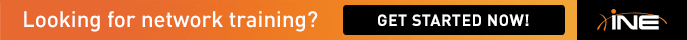# Catalyst QoS - Ingress queueing clarification

CCIE Routing and Switching V4 Cisco Press says:

When QoS is enabled on a Cisco 3560 switch, the default ingress queue settings are as follows:

• Queue 2 is the priority queue.

• Queue 2 is allocated 10 percent of the interface bandwidth.

• CoS 5 traffic is placed in queue 2.

But also says:

By Default: Queue 1= 90% Buffers ; Queue 2=10% Buffers

Bandwidth Weighing Queue 1=4 ; Queue 2=4.

It appears the relative Bandwidth Weightings are equal(how frequently scheduler pulls from buffers) but because Queue1 has 90% buffers and Queue2 10% buffers.... consequently Queue 1 ends up consuming 90% of interface bandwidth and Queue 2 10%.

Is that interpretation correct?

## Comments

• I think priority queue is given 10% bandwidth initially because it's prioritized & rest of the bandwidth is used by other queues. So, PQ only have 10% queue & checked first. Correct me if i'm wrong.

• Hari,

Thanks. True Queue 2 is by default Priority and gets 10% and is checked first.

However there are two parts: 1. Priority Queue reservation 10% 2. The rest of traffic(90%) split between both Queues 1 and 2.

So by default Queue 2 gets: 10% + [10/100*90]=19% Total Interface bandwidth on average....

Below is elaboration of the Part 2 above(Non-Priority or Non-Reserved part of the traffic)

1. Buffers(Memory) and 2. Bandwidth Weighting(frequency of grabbing buffer contents and putting on the line) and the frequency is equally divided 4:4 between Queue 1 and Queue 2.

Queue 2(Priority Queue) gets its 10% and Queue 1(non-priority Queue) gets its 90% buffer put on the line....so pass 1 10% Queue 2-> pass 2 90% Queue 1 and pass1+2 makes 1 cycle...etc...resulting in

Queue 2 getting 1/10 or 10% of NON-reserved interface bandwidth

Then suppose Bandwidth weightings are changed from 4:4 to 2:1 and buffers still remain at 90% Queue 1 to 10% Queue 2

2 of Queue1 : 1 of Queue2.  So 1 cycle [10% Queue2 {1/10} => 90% Queue1{9/10} =>90%Queue1{9/10} OR 1/10 : 18/10=>1:18

Queue2 is 1/19*100=5.26% of NON-Reserved Interface bandwidth when weighting is 2:1 between Queue 1 and 2 respectively

Is this reasoning correct on the relationship between  reserved bandwidth, buffers and bandwidth weights?

Sign In or Register to comment.